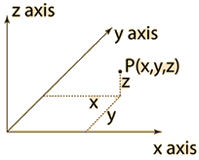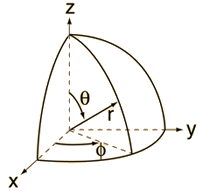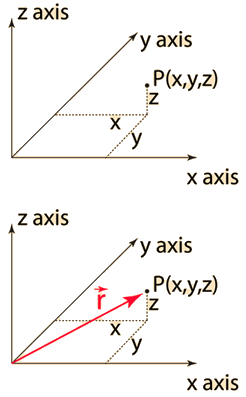# Coordinate Systems

 Rectangular Coordinates Cylindrical Polar Coordinates Spherical Polar CoordinatesApplications:Distance between points Applications:Cylindrical capacitorElectric field of line charge. Applications:Hydrogen Schrodinger EquationMaxwell speed distributionElectric potential of sphere
Index

 HyperPhysics****HyperMath*****Geometry R Nave
Go Back

# Rectangular Coordinates

The most common coordinate system for representing positions in space is one based on three perpendicular spatial axes generally designated x, y, and z.Any point P may be represented by three signed numbers, usually written (x, y, z) where the coordinate is the perpendicular distance from the plane formed by the other two axes. Often positions are specified by a position vector r which can be expressed in terms of the coordinate values and associated unit vectors.Although the entire coordinate system can be rotated, the relationship between the axes is fixed in what is called a right-handed coordinate system. For the display of some kinds of data,it may be convenient to have different scales for the different axes, but for the purpose of mathematical operations with the coordinates, it is necessary for the axes to have the same scales. The term "Cartesian coordinates" is used to describe such systems, and the values of the three coordinates unambiguously locate a point in space. In such a coordinate system you can calculate the distance between two points and perform operations like axis rotations without altering this value. The distance between any two points in rectangular coordinates can be found from the distance relationship.

### Operations in Cartesian coordinates.

Index

 HyperPhysics****HyperMath*****Geometry R Nave
Go Back

# Operations in Cartesian Coordinates

Distance between pointsVector calculus operations